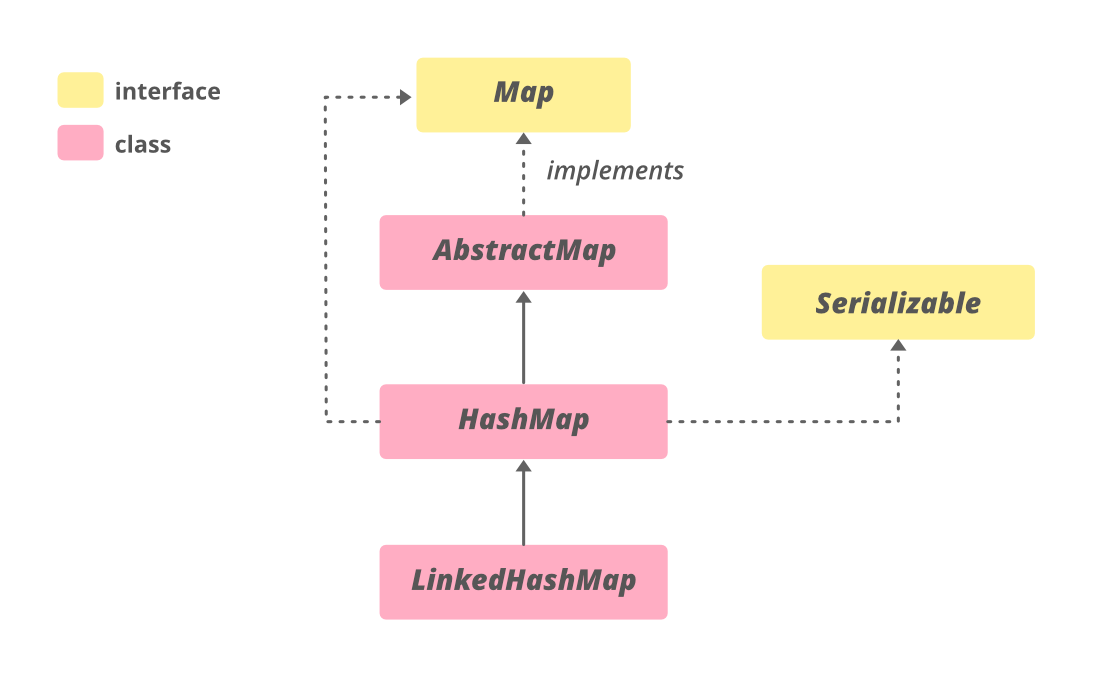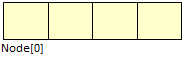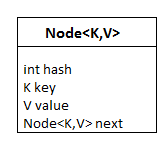GeeksforGeeks App
Open AppBrowser
Continue

# HashMap in Java

In Java, HashMap is a part of Java’s collection since Java 1.2. This class is found in java.util package. It provides the basic implementation of the Map interface of Java. HashMap in Java stores the data in (Key, Value) pairs, and you can access them by an index of another type (e.g. an Integer). One object is used as a key (index) to another object (value). If you try to insert the duplicate key in HashMap, it will replace the element of the corresponding key.

## What is HashMap?

Java HashMap is similar to HashTable, but it is unsynchronized. It allows to store the null keys as well, but there should be only one null key object and there can be any number of null values. This class makes no guarantees as to the order of the map. To use this class and its methods, you need to import java.util.HashMap package or its superclass.

## Java

 `// Java program to illustrate HashMap class of java.util``// package`` ` `// Importing HashMap class``import` `java.util.HashMap;`` ` `// Main class``public` `class` `GFG {`` ` `    ``// Main driver method``    ``public` `static` `void` `main(String[] args)``    ``{``        ``// Create an empty hash map by declaring object``        ``// of string and integer type``        ``HashMap map = ``new` `HashMap<>();`` ` `        ``// Adding elements to the Map``        ``// using standard put() method``        ``map.put(``"vishal"``, ``10``);``        ``map.put(``"sachin"``, ``30``);``        ``map.put(``"vaibhav"``, ``20``);`` ` `        ``// Print size and content of the Map``        ``System.out.println(``"Size of map is:- "``                           ``+ map.size());`` ` `        ``// Printing elements in object of Map``        ``System.out.println(map);`` ` `        ``// Checking if a key is present and if``        ``// present, print value by passing``        ``// random element``        ``if` `(map.containsKey(``"vishal"``)) {`` ` `            ``// Mapping``            ``Integer a = map.get(``"vishal"``);`` ` `            ``// Printing value for the corresponding key``            ``System.out.println(``"value for key"``                               ``+ ``" \"vishal\" is:- "` `+ a);``        ``}``    ``}``}`

Output

```Size of map is:- 3
{vaibhav=20, vishal=10, sachin=30}
value for key "vishal" is:- 10```

## HashMap Declaration

```public class HashMap<K,V> extends AbstractMap<K,V>
implements Map<K,V>, Cloneable, Serializable```

### Parameters:

It takes two parameters namely as follows:

• The type of keys maintained by this map
• The type of mapped values

HashMap in Java implements Serializable, Cloneable, Map<K, V> interfaces.Java HashMap extends AbstractMap<K, V> class. The direct subclasses are LinkedHashMap and PrinterStateReasons.

## Hierarchy of Java HashMap in Java### Characteristics of HashMap:

A HashMap is a data structure that is used to store and retrieve values based on keys. Some of the key characteristics of a hashmap include:

• Fast access time: HashMaps provide constant time access to elements, which means that retrieval and insertion of elements are very fast, usually O(1) time complexity.
• Uses hashing function: HashMaps uses a hash function to map keys to indices in an array. This allows for a quick lookup of values based on keys.
• Stores key-value pairs: Each element in a HashMap consists of a key-value pair. The key is used to look up the associated value.
• Supports null keys and values: HashMaps allow for null values and keys. This means that a null key can be used to store a value, and a null value can be associated with a key.
• Not ordered: HashMaps are not ordered, which means that the order in which elements are added to the map is not preserved. However, LinkedHashMap is a variation of HashMap that preserves the insertion order.
• Allows duplicates: HashMaps allow for duplicate values, but not duplicate keys. If a duplicate key is added, the previous value associated with the key is overwritten.
• Thread-unsafe: HashMaps are not thread-safe, which means that if multiple threads access the same hashmap simultaneously, it can lead to data inconsistencies. If thread safety is required, ConcurrentHashMap can be used.
• Capacity and load factor: HashMaps have a capacity, which is the number of elements that it can hold, and a load factor, which is the measure of how full the hashmap can be before it is resized.

## Java

 `import` `java.util.HashMap;`` ` `public` `class` `ExampleHashMap {``   ``public` `static` `void` `main(String[] args) {``       ` `      ``// Create a HashMap``      ``HashMap hashMap = ``new` `HashMap<>();``       ` `      ``// Add elements to the HashMap``      ``hashMap.put(``"John"``, ``25``);``      ``hashMap.put(``"Jane"``, ``30``);``      ``hashMap.put(``"Jim"``, ``35``);``       ` `      ``// Access elements in the HashMap``      ``System.out.println(hashMap.get(``"John"``)); ``// Output: 25``       ` `      ``// Remove an element from the HashMap``      ``hashMap.remove(``"Jim"``);``       ` `      ``// Check if an element is present in the HashMap``      ``System.out.println(hashMap.containsKey(``"Jim"``)); ``// Output: false``       ` `      ``// Get the size of the HashMap``      ``System.out.println(hashMap.size()); ``// Output: 2``   ``}``}`

Output

```25
false
2```

## Constructors in HashMap

HashMap provides 4 constructors and the access modifier of each is public which are listed as follows:

1. HashMap()
2. HashMap(int initialCapacity)
4. HashMap(Map map)

Now discussing the above constructors one by one alongside implementing the same with help of clean Java programs.

### 1. HashMap()

It is the default constructor which creates an instance of HashMap with an initial capacity of 16 and a load factor of 0.75.

Syntax:

`HashMap<K, V> hm = new HashMap<K, V>();`

Example

## Java

 `// Java program to Demonstrate the HashMap() constructor`` ` `// Importing basic required classes``import` `java.io.*;``import` `java.util.*;`` ` `// Main class``// To add elements to HashMap``class` `GFG {`` ` `    ``// Main driver method``    ``public` `static` `void` `main(String args[])``    ``{``        ``// No need to mention the``        ``// Generic type twice``        ``HashMap hm1 = ``new` `HashMap<>();`` ` `        ``// Initialization of a HashMap using Generics``        ``HashMap hm2``            ``= ``new` `HashMap();`` ` `        ``// Adding elements using put method``        ``// Custom input elements``        ``hm1.put(``1``, ``"one"``);``        ``hm1.put(``2``, ``"two"``);``        ``hm1.put(``3``, ``"three"``);`` ` `        ``hm2.put(``4``, ``"four"``);``        ``hm2.put(``5``, ``"five"``);``        ``hm2.put(``6``, ``"six"``);`` ` `        ``// Print and display mapping of HashMap 1``        ``System.out.println(``"Mappings of HashMap hm1 are : "``                           ``+ hm1);`` ` `        ``// Print and display mapping of HashMap 2``        ``System.out.println(``"Mapping of HashMap hm2 are : "``                           ``+ hm2);``    ``}``}`

Output

```Mappings of HashMap hm1 are : {1=one, 2=two, 3=three}
Mapping of HashMap hm2 are : {4=four, 5=five, 6=six}```

### 2. HashMap(int initialCapacity)

It creates a HashMap instance with a specified initial capacity and load factor of 0.75.

Syntax:

`HashMap<K, V> hm = new HashMap<K, V>(int initialCapacity);`

Example

## Java

 `// Java program to Demonstrate``// HashMap(int initialCapacity) Constructor`` ` `// Importing basic classes``import` `java.io.*;``import` `java.util.*;`` ` `// Main class``// To add elements to HashMap``class` `AddElementsToHashMap {`` ` `    ``// Main driver method``    ``public` `static` `void` `main(String args[])``    ``{``        ``// No need to mention the``        ``// Generic type twice``        ``HashMap hm1 = ``new` `HashMap<>(``10``);`` ` `        ``// Initialization of a HashMap using Generics``        ``HashMap hm2``            ``= ``new` `HashMap(``2``);`` ` `        ``// Adding elements to object of HashMap``        ``// using put method`` ` `        ``// HashMap 1``        ``hm1.put(``1``, ``"one"``);``        ``hm1.put(``2``, ``"two"``);``        ``hm1.put(``3``, ``"three"``);`` ` `        ``// HashMap 2``        ``hm2.put(``4``, ``"four"``);``        ``hm2.put(``5``, ``"five"``);``        ``hm2.put(``6``, ``"six"``);`` ` `        ``// Printing elements of HashMap 1``        ``System.out.println(``"Mappings of HashMap hm1 are : "``                           ``+ hm1);`` ` `        ``// Printing elements of HashMap 2``        ``System.out.println(``"Mapping of HashMap hm2 are : "``                           ``+ hm2);``    ``}``}`

Output

```Mappings of HashMap hm1 are : {1=one, 2=two, 3=three}
Mapping of HashMap hm2 are : {4=four, 5=five, 6=six}```

### 3. HashMap(int initialCapacity, float loadFactor)

It creates a HashMap instance with a specified initial capacity and specified load factor.

Syntax:

`HashMap<K, V> hm = new HashMap<K, V>(int initialCapacity, float loadFactor);`

Example

## Java

 `// Java program to Demonstrate``// HashMap(int initialCapacity,float loadFactor) Constructor`` ` `// Importing basic classes``import` `java.io.*;``import` `java.util.*;`` ` `// Main class``// To add elements to HashMap``class` `GFG {`` ` `    ``// Main driver method``    ``public` `static` `void` `main(String args[])``    ``{``        ``// No need to mention the generic type twice``        ``HashMap hm1``            ``= ``new` `HashMap<>(``5``, ``0``.75f);`` ` `        ``// Initialization of a HashMap using Generics``        ``HashMap hm2``            ``= ``new` `HashMap(``3``, ``0``.5f);`` ` `        ``// Add Elements using put() method``        ``// Custom input elements``        ``hm1.put(``1``, ``"one"``);``        ``hm1.put(``2``, ``"two"``);``        ``hm1.put(``3``, ``"three"``);`` ` `        ``hm2.put(``4``, ``"four"``);``        ``hm2.put(``5``, ``"five"``);``        ``hm2.put(``6``, ``"six"``);`` ` `        ``// Print and display elements in object of hashMap 1``        ``System.out.println(``"Mappings of HashMap hm1 are : "``                           ``+ hm1);`` ` `        ``// Print and display elements in object of hashMap 2``        ``System.out.println(``"Mapping of HashMap hm2 are : "``                           ``+ hm2);``    ``}``}`

Output

```Mappings of HashMap hm1 are : {1=one, 2=two, 3=three}
Mapping of HashMap hm2 are : {4=four, 5=five, 6=six}```

### 4. HashMap(Map map)

It creates an instance of HashMap with the same mappings as the specified map.

HashMap<K, V> hm = new HashMap<K, V>(Map map);

## Java

 `// Java program to demonstrate the ``// HashMap(Map map) Constructor`` ` `import` `java.io.*;``import` `java.util.*;`` ` `class` `AddElementsToHashMap {``    ``public` `static` `void` `main(String args[])``    ``{``        ``// No need to mention the``        ``// Generic type twice``        ``Map hm1 = ``new` `HashMap<>();`` ` `        ``// Add Elements using put method``        ``hm1.put(``1``, ``"one"``);``        ``hm1.put(``2``, ``"two"``);``        ``hm1.put(``3``, ``"three"``);`` ` `        ``// Initialization of a HashMap``        ``// using Generics``        ``HashMap hm2``            ``= ``new` `HashMap(hm1);`` ` `        ``System.out.println(``"Mappings of HashMap hm1 are : "``                           ``+ hm1);``       ` `        ``System.out.println(``"Mapping of HashMap hm2 are : "``                           ``+ hm2);``    ``}``}`

Output

```Mappings of HashMap hm1 are : {1=one, 2=two, 3=three}
Mapping of HashMap hm2 are : {1=one, 2=two, 3=three}```

## Performing Various Operations on HashMap

In order to add an element to the map, we can use the put() method. However, the insertion order is not retained in the Hashmap. Internally, for every element, a separate hash is generated and the elements are indexed based on this hash to make it more efficient.

## Java

 `// Java program to add elements``// to the HashMap`` ` `import` `java.io.*;``import` `java.util.*;`` ` `class` `AddElementsToHashMap {``    ``public` `static` `void` `main(String args[])``    ``{``        ``// No need to mention the``        ``// Generic type twice``        ``HashMap hm1 = ``new` `HashMap<>();`` ` `        ``// Initialization of a HashMap``        ``// using Generics``        ``HashMap hm2``            ``= ``new` `HashMap();`` ` `        ``// Add Elements using put method``        ``hm1.put(``1``, ``"Geeks"``);``        ``hm1.put(``2``, ``"For"``);``        ``hm1.put(``3``, ``"Geeks"``);`` ` `        ``hm2.put(``1``, ``"Geeks"``);``        ``hm2.put(``2``, ``"For"``);``        ``hm2.put(``3``, ``"Geeks"``);`` ` `        ``System.out.println(``"Mappings of HashMap hm1 are : "``                           ``+ hm1);``        ``System.out.println(``"Mapping of HashMap hm2 are : "``                           ``+ hm2);``    ``}``}`

Output

```Mappings of HashMap hm1 are : {1=Geeks, 2=For, 3=Geeks}
Mapping of HashMap hm2 are : {1=Geeks, 2=For, 3=Geeks}```

### 2. Changing Elements

After adding the elements if we wish to change the element, it can be done by again adding the element with the put() method. Since the elements in the map are indexed using the keys, the value of the key can be changed by simply inserting the updated value for the key for which we wish to change.

## Java

 `// Java program to change``// elements of HashMap`` ` `import` `java.io.*;``import` `java.util.*;``class` `ChangeElementsOfHashMap {``    ``public` `static` `void` `main(String args[])``    ``{`` ` `        ``// Initialization of a HashMap``        ``HashMap hm``            ``= ``new` `HashMap();`` ` `        ``// Change Value using put method``        ``hm.put(``1``, ``"Geeks"``);``        ``hm.put(``2``, ``"Geeks"``);``        ``hm.put(``3``, ``"Geeks"``);`` ` `        ``System.out.println(``"Initial Map "` `+ hm);`` ` `        ``hm.put(``2``, ``"For"``);`` ` `        ``System.out.println(``"Updated Map "` `+ hm);``    ``}``}`

Output

```Initial Map {1=Geeks, 2=Geeks, 3=Geeks}
Updated Map {1=Geeks, 2=For, 3=Geeks}```

### 3. Removing Element

In order to remove an element from the Map, we can use the remove() method. This method takes the key value and removes the mapping for a key from this map if it is present in the map.

## Java

 `// Java program to remove``// elements from HashMap`` ` `import` `java.io.*;``import` `java.util.*;``class` `RemoveElementsOfHashMap{``    ``public` `static` `void` `main(String args[])``    ``{``        ``// Initialization of a HashMap``        ``Map hm``            ``= ``new` `HashMap();`` ` `        ``// Add elements using put method``        ``hm.put(``1``, ``"Geeks"``);``        ``hm.put(``2``, ``"For"``);``        ``hm.put(``3``, ``"Geeks"``);``        ``hm.put(``4``, ``"For"``);`` ` `        ``// Initial HashMap``        ``System.out.println(``"Mappings of HashMap are : "``                           ``+ hm);`` ` `        ``// remove element with a key``        ``// using remove method``        ``hm.remove(``4``);`` ` `        ``// Final HashMap``        ``System.out.println(``"Mappings after removal are : "``                           ``+ hm);``    ``}``}`

Output

```Mappings of HashMap are : {1=Geeks, 2=For, 3=Geeks, 4=For}
Mappings after removal are : {1=Geeks, 2=For, 3=Geeks}```

### 4. Traversal of HashMap

We can use the Iterator interface to traverse over any structure of the Collection Framework. Since Iterators work with one type of data we use Entry< ? , ? > to resolve the two separate types into a compatible format. Then using the next() method we print the entries of HashMap.

## Java

 `// Java program to traversal a``// Java.util.HashMap`` ` `import` `java.util.HashMap;``import` `java.util.Map;`` ` `public` `class` `TraversalTheHashMap {``    ``public` `static` `void` `main(String[] args)``    ``{``        ``// initialize a HashMap``        ``HashMap map = ``new` `HashMap<>();`` ` `        ``// Add elements using put method``        ``map.put(``"vishal"``, ``10``);``        ``map.put(``"sachin"``, ``30``);``        ``map.put(``"vaibhav"``, ``20``);`` ` `        ``// Iterate the map using``        ``// for-each loop``        ``for` `(Map.Entry e : map.entrySet())``            ``System.out.println(``"Key: "` `+ e.getKey()``                               ``+ ``" Value: "` `+ e.getValue());``    ``}``}`

Output

```Key: vaibhav Value: 20
Key: vishal Value: 10
Key: sachin Value: 30```

## Important Features of HashMap

To access a value one must know its key. HashMap is known as HashMap because it uses a technique called Hashing. Hashing is a technique of converting a large String to a small String that represents the same String. A shorter value helps in indexing and faster searches. HashSet also uses HashMap internally.
A few important features of HashMap are:

• HashMap is a part of java.util package.
• HashMap extends an abstract class AbstractMap which also provides an incomplete implementation of the Map interface.
• It also implements a Cloneable and Serializable interfaces. K and V in the above definition represent Key and Value respectively.
• HashMap doesn’t allow duplicate keys but allows duplicate values. That means A single key can’t contain more than 1 value but more than 1 key can contain a single value.
• HashMap allows a null key also but only once and multiple null values.
• This class makes no guarantees as to the order of the map; in particular, it does not guarantee that the order will remain constant over time. It is roughly similar to HashTable but is unsynchronized.

## Internal Structure of HashMap

Internally HashMap contains an array of Node and a node is represented as a class that contains 4 fields:

1. int hash
2. K key
3. V value
4. Node next

It can be seen that the node is containing a reference to its own object. So it’s a linked list.

HashMap:Node:## Performance of HashMap

The performance of HashMap depends on 2 parameters which are named as follows:

1. Initial Capacity

1. Initial Capacity – It is the capacity of HashMap at the time of its creation (It is the number of buckets a HashMap can hold when the HashMap is instantiated). In java, it is 2^4=16 initially, meaning it can hold 16 key-value pairs.

2. Load Factor – It is the percent value of the capacity after which the capacity of Hashmap is to be increased (It is the percentage fill of buckets after which Rehashing takes place). In java, it is 0.75f by default, meaning the rehashing takes place after filling 75% of the capacity.

3. Threshold – It is the product of Load Factor and Initial Capacity. In java, by default, it is (16 * 0.75 = 12). That is, Rehashing takes place after inserting 12 key-value pairs into the HashMap.

4. Rehashing – It is the process of doubling the capacity of the HashMap after it reaches its Threshold. In java, HashMap continues to rehash(by default) in the following sequence – 2^4, 2^5, 2^6, 2^7, …. so on.

If the initial capacity is kept higher then rehashing will never be done. But by keeping it higher increases the time complexity of iteration. So it should be chosen very cleverly to increase performance. The expected number of values should be taken into account to set the initial capacity. The most generally preferred load factor value is 0.75 which provides a good deal between time and space costs. The load factor’s value varies between 0 and 1.

Note: From Java 8 onward, Java has started using Self Balancing BST instead of a linked list for chaining. The advantage of self-balancing bst is, we get the worst case (when every key maps to the same slot) search time is O(Log n).

## Synchronized HashMap

As it is told that HashMap is unsynchronized i.e. multiple threads can access it simultaneously. If multiple threads access this class simultaneously and at least one thread manipulates it structurally then it is necessary to make it synchronized externally. It is done by synchronizing some object which encapsulates the map. If No such object exists then it can be wrapped around Collections.synchronizedMap() to make HashMap synchronized and avoid accidental unsynchronized access. As in the following example:

`Map m = Collections.synchronizedMap(new HashMap(...));`

Now the Map m is synchronized.  Iterators of this class are fail-fast if any structure modification is done after the creation of the iterator, in any way except through the iterator’s remove method. In a failure of an iterator, it will throw ConcurrentModificationException.

The time complexity of HashMap:

HashMap provides constant time complexity for basic operations, get and put if the hash function is properly written and it disperses the elements properly among the buckets. Iteration over HashMap depends on the capacity of HashMap and the number of key-value pairs. Basically, it is directly proportional to the capacity + size. Capacity is the number of buckets in HashMap. So it is not a good idea to keep a high number of buckets in HashMap initially.

Applications of HashMap:

HashMap is mainly the implementation of hashing. It is useful when we need efficient implementation of search, insert and delete operations. Please refer to the applications of hashing for details.

## Methods in HashMapassociate

• K – The type of the keys in the map.
• V – The type of values mapped in the map.

### Methods inherited from interface java.util.Map

• Fast retrieval: HashMaps provide constant time access to elements, which means that retrieval and insertion of elements is very fast.
• Efficient storage: HashMaps use a hashing function to map keys to indices in an array. This allows for quick lookup of values based on keys, and efficient storage of data.
• Flexibility: HashMaps allow for null keys and values, and can store key-value pairs of any data type.
• Easy to use: HashMaps have a simple interface and can be easily implemented in Java.
• Suitable for large data sets: HashMaps can handle large data sets without slowing down.

• Unordered: HashMaps are not ordered, which means that the order in which elements are added to the map is not preserved.
• Not thread-safe: HashMaps are not thread-safe, which means that if multiple threads access the same hashmap simultaneously, it can lead to data inconsistencies.
• Performance can degrade: In some cases, if the hashing function is not properly implemented or if the load factor is too high, the performance of a HashMap can degrade.
• More complex than arrays or lists: HashMaps can be more complex to understand and use than simple arrays or lists, especially for beginners.
• Higher memory usage: Since HashMaps use an underlying array, they can use more memory than other data structures like arrays or lists. This can be a disadvantage if memory usage is a concern.

## FAQs in HashMap

### Q1. What is a HashMap in Java?

HashMap in Java is the class from the collection framework that can store key-value pairs inside it.

### Q2. Why use HashMap in Java?

HashMap in Java is used for storing key-value pairs where each key is unique.

### Q3. What is the benefit of HashMap?

HashMap is used because of it provides features like:

• Fast retrieval
• Efficient storage
• Flexible to use
• Easy to use
• Suitable for large data sets